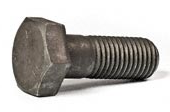Engineering ToolBox - Resources, Tools and Basic Information for Engineering and Design of Technical Applications!

# Bolt Stretching and Tensile Stress

## Tensile stress and Hooke's Law.### Elongation

Bolt stretching according Hook's Law can be calculated as

dl = F L / E A                      (1)

where

dl = change in length of bolt (inches, m)

F = applied tensile load (lb, N)

L = effective length of bolt where tensile strength is applied (inches, m)

E = Young's Modulus of Elasticity (psi, N/m2)

A = tensile stress area of the bolt (square inches, m2)

### Stress Area

The tensile stress area can be calculated as

A = (π / 4) (d - 0.9743 / n)2                       (2)

where

d = nominal diameter of bolt (in)

n = 1 / p = number of threads per inch

p = pitch, length per thread (in)

### Tensile Stress

Tensile stress can be calculated as

σ = F / A                      (3)

where

σ = tensile stress (psi, N/m2 (Pa))

### Example - Bolt Stretching - Imperial Units

• stud diameter : 7/8 inches
• Young's Modulus steel : 30 106 psi
• designed bolt load : 10000 lb
• effective length : 5 inches

The tensile stress area can be calculated as

A = 0.7854 ((7/8 in) - 0.9743 / 9)2

= 0.46 (in2)

The elongation can be calculated as

dl = (10000 lb) (5 in) / ((30 106 psi) (0.46 in2))

= 0.0036 (inches)

The tensile stress can be calculated as

σ = (10000 lb) / (0.46 in2)

= 21740 psi

## Related Topics

• Fasteners - Bolts, nuts and threaded rods - torque, tension and loads.

## Engineering ToolBox - SketchUp Extension - Online 3D modeling!

Add standard and customized parametric components - like flange beams, lumbers, piping, stairs and more - to your Sketchup model with the Engineering ToolBox - SketchUp Extension - enabled for use with the amazing, fun and free SketchUp Make and SketchUp Pro .Add the Engineering ToolBox extension to your SketchUp from the SketchUp Pro Sketchup Extension Warehouse!

Translate

## Privacy

We don't collect information from our users. Only emails and answers are saved in our archive. Cookies are only used in the browser to improve user experience.

Some of our calculators and applications let you save application data to your local computer. These applications will - due to browser restrictions - send data between your browser and our server. We don't save this data.

## Citation

• Engineering ToolBox, (2008). Bolt Stretching and Tensile Stress. [online] Available at: https://www.engineeringtoolbox.com/bolt-stretching-d_1164.html [Accessed Day Mo. Year].

Modify access date.

. .

#### Scientific Online Calculator4 29